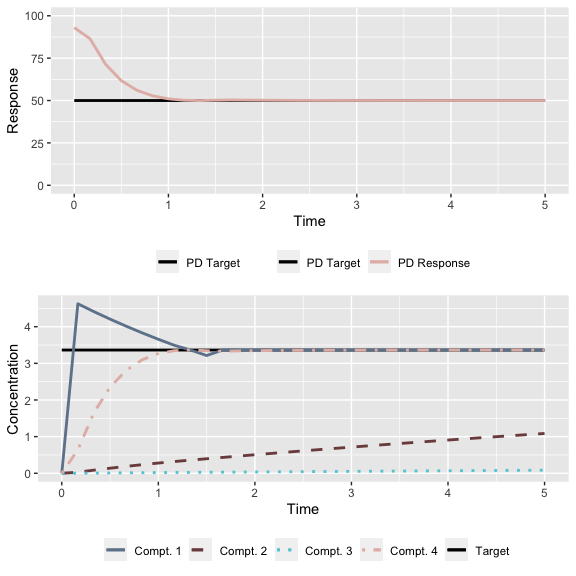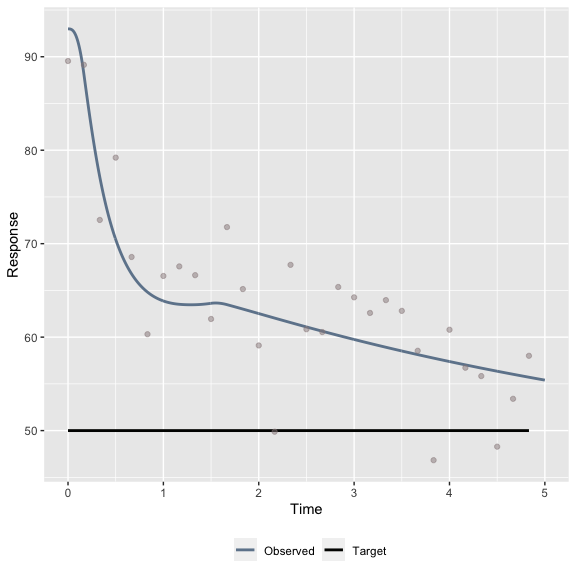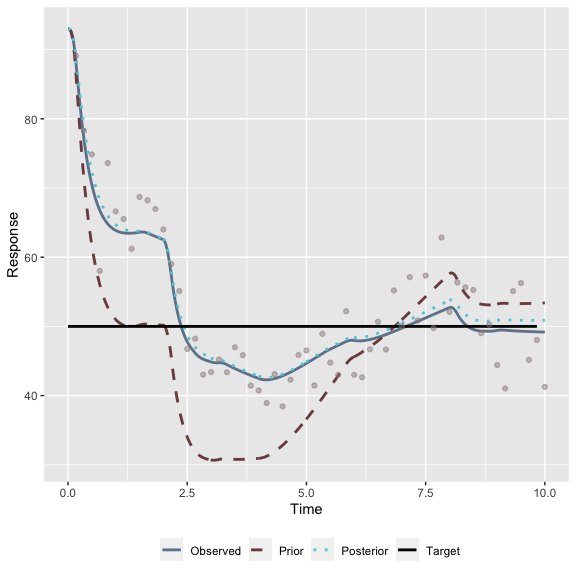# Open- and closed-loop control with tci

Target-controlled infusion (TCI) systems are commonly used to implement open- and closed-loop control over drug delivery to a patient. In open-loop control (OLC), the TCI system delivers medication to maintain a target effect or concentration set by a clinician based on a pre-specified patient pharamacokinetic (PK) or pharmacokinetic-pharmacodynamic (PK-PD) model. Typically, these models will be adjusted for patient covariate values (e.g. age, weight) and the clinician may modify the target as needed based on observed responses. In closed-loop control (CLC) systems, patient data collected in real-time are collected and used to titrate infusion rates through a feedback mechanism. For some control systems, such as proportional-integral-derivative (PID) controllers, the difference between observed values and a setpoint is used as an input into a pre-tuned controller that modifies the infusion rate directly. Bayesian controllers, by contrast, update an objective function (i.e. the posterior distribution of PK or PK-PD model parameters for a particular patient) using the observed data and recalculate infusion rates based on the updated model.

In this vignette, we demonstrate how OLC and Bayesian CLC can be implemented using the tci package. We illustrate this using the Eleveld et al. (2018) PK-PD model for the anesthetic propofol. The Eleveld model is a three-compartment model with an additional effect-site that is linked to an Emax PD model that describes the behavior of patient Bispectral Index (BIS) values as a function of effect-site concentration. BIS is a unitless, EEG-derived index with values between zero and 100 with lower values indicating greater degrees of sedation. For general anesthesia, a fixed set-point of BIS = 50 is commonly used with values between 40 and 60 considered in-range.(Brogi et al. 2017) PK parameters are adjusted for patient age, post-menstrual age (PMA), weight, height, BMI, sex, and use of concomitant opioids or local anesthetic drugs, while PD parameters are adjusted for patient age with an additional age-based time lag in BIS measurements.

The Eleveld PK-PD model was developed from 30 prior studies, five of which contained BIS measurements in addition to plasma propofol concentrations. These study data used to fit the model have generously been made available by Eleveld et al. and their respective authors, largely through the “Open TCI” initiative http://opentci.org/. The data used to fit the Eleveld model has generously been made available by the authors, as well as the authors of the constituent studies. Within the tci package, the datasets eleveld_pk and eleveld_pd contain the post-model-fitting empirical Bayes (EB) estimates of PK-PD parameters for each of the patients, as well as patient covariate values. The EB estimates represent the set of highest-probability (i.e. posterior mode) parameter values for each patient and, if desired, can be used in simulations as a set of “true” but unobserved patient parameter values.

library(tci)

data("eleveld_pk")
data("eleveld_pd")

# 122 patients had both pk and pd measurements
pkpd_eb <- merge(eleveld_pk, eleveld_pd)

### Open-loop control

For each patient, the prior, covariate-based parameter estimates can be calculated using the eleveld_poppk function. The df argument should contain a data frame with columns specifying each of the covariate values used in evaluating the PK-PD model. For the Eleveld model, these are “AGE,” “PMA,” “WGT,” “HGT,” “M1F2,” “TECH,” and “A1V2.” The argument rate is used to specify if elimination rate constants should be returned in addition to clearance parameters. The rand argument can be used to draw Monte-Carlo estimates from the population at the specified covariate values based on unexplained population PK-PD variability.

Using Patient 1, we can identify an infusion schedule aimed at targeting a BIS value of 50.

# Evaluate Eleveld model at covariate values for patient 1
prior_pars_id1 <- eleveld_poppk(df = pkpd_eb[1,])
prior_pars_id1
#>    ID AGE WGT HGT M1F2   PMA TECH A1V2       V1       V2       V3     CL
#> 1 403   5  15 107    2 5.769    1    2 5.684869 8.725357 61.99246 0.6614
#>          Q2        Q3    BMI   FFM   E50      KE0   EMAX    GAM   GAM1  RESD
#> 1 0.6607379 0.3083446 13.102 12.37 2.561 1.822525 92.982 1.4736 1.8939 5.489
#>     ALAG1 LN_SIGMA     CE50 BIS0 GAMMA GAMMA2 SIGMA BIS_DELAY
#> 1 0.27159    0.191 3.726351   93  1.47   1.89  8.03  16.29499

# tci infusions are calculated using prior parameter estimates
pars_pk_id1 <- unlist(prior_pars_id1[,c("V1","V2","V3","CL","Q2","Q3","KE0")])
# BIS0 is repeated since BIS at maximum effect = BIS0 for Eleveld model
pars_pd_id1 <- unlist(prior_pars_id1[,c("CE50","GAMMA","GAMMA2","BIS0","BIS0")])

The tci_pd function extends the tci function to PK-PD models and requires the specification of functions evaluating the PD model and its inverse, as well as parameter values for the PK-PD functions. The plot.tciinf S3 method can be used to visualize the results.

olc_inf_id1 <- tci_pd(pdresp = c(50,50), # target BIS = 50
tms = c(0,5), # target for 5 minutes
pkmod = pkmod3cptm, # pk model
pdmod = emax_eleveld, # pd model
pdinv = inv_emax_eleveld, # inverse pd model
pars_pk = pars_pk_id1,
pars_pd = pars_pd_id1)
plot(olc_inf_id1)The plotted values represent how the patient is expected to respond to the specified infusion schedule if their true underlying PK-PD parameters are identical to those predicted at their covariate values. To evaluate how this patient would respond to this infusion at a different set of “true” parameters, we can simulate data using the infusion schedule specified in the object olc_inf_id1 at alternate parameters.

# true set of patient parameters given by EB estimates
eb_pars_pk_id1 <- unlist(pkpd_eb[1,c("CL","Q2","Q3","V1","V2","V3","KE0")])
eb_pars_pd_id1 <- unlist(pkpd_eb[1,c("E50","GAM","GAM1","EMAX","EMAX")])

# residual error term
eb_sigma_id1 <- unlist(pkpd_eb[1,"RESD"])

# BIS delay - convert from minutes to seconds
eb_bd_id1 <- unlist(pkpd_eb[1,"ALAG1"]) * 60

# simulate response to infusions
set.seed(1)
datasim_id1 <- gen_data(inf = olc_inf_id1,
pkmod = pkmod3cptm,
pdmod = emax_eleveld,
pars_pk0 = eb_pars_pk_id1,
pars_pd0 = eb_pars_pd_id1,
delay = eb_bd_id1)

# plot results
plot(datasim_id1)### Closed-loop control

Closed-loop control can be implemented within the tci package through Bayesian updates to the patient-specific PK-PD model using the function bayes_control. bayes_control requires four components as arguments: 1) a data frame specifying a set of targets and corresponding times, 2) a data frame specifying update times, 3) a set of prior PK-PD estimates, and 4) a set of true PK-PD values.

For the targets, we target BIS = 50 for a 10-minute period with PK-PD parameters updated every two minutes. The full_data argument in the updates data frame specifies if all available data should be used at each update, rather than just the data collected since the prior update. Setting full_data = TRUE will result in more accurate estimates at the expense of additional computational time. When specifying prior parameter values, we need to identify a variance-covariance matrix that can be updated as data are collected. The eleveld_vcov function estimates the variance-covariance matrix conditioned on an individual’s covariates by randomly sampling from and averaging over the distribution of random effects.

cl_targets <- function(time, target){
data.frame(time = time, target = target)
}

cl_updates <- function(time, full_data = TRUE, plot_progress = FALSE){
data.frame(time = time, full_data = full_data, plot_progress = plot_progress)
}

# 1) Data frame of targets
targets = cl_targets(time = seq(0,10,1/6),
target = 50)

# 2) Update times

# 3) prior parameter values based on point estimates at covariate values
prior_par_list <- list(
pars_pkpd = prior_pars_id1[c("CL","Q2","Q3","V1","V2","V3",
"KE0","CE50","GAMMA","GAMMA2",
"BIS0","BIS0")],
pk_ix = 1:7, # indices of PK parameters
pd_ix = 8:12,
fixed_ix = 9:12, # indices of parameters that aren't updated
err = prior_pars_id1[1,"SIGMA"], # residual error
sig = eleveld_vcov(prior_pars_id1,
rates = FALSE)[]
)

# 4) true parameter values based on EB values
true_par_list <- list(pars_pkpd = pkpd_eb[1,c("CL","Q2","Q3","V1",
"V2","V3","KE0",
"E50","GAM","GAM1",
"EMAX","EMAX")],
pk_ix = 1:7,
pd_ix = 8:12,
fixed_ix = 9:12,
err = pkpd_eb[1,"RESD"],
delay = pkpd_eb[1,"ALAG1"]) 

The bayes_control function runs the closed-loop simulation and can be plotted using the plot.bayessim S3 method.

# run simulation
bayes_sim <- bayes_control(targets = targets,
plot(bayes_sim)